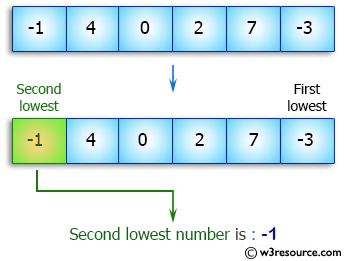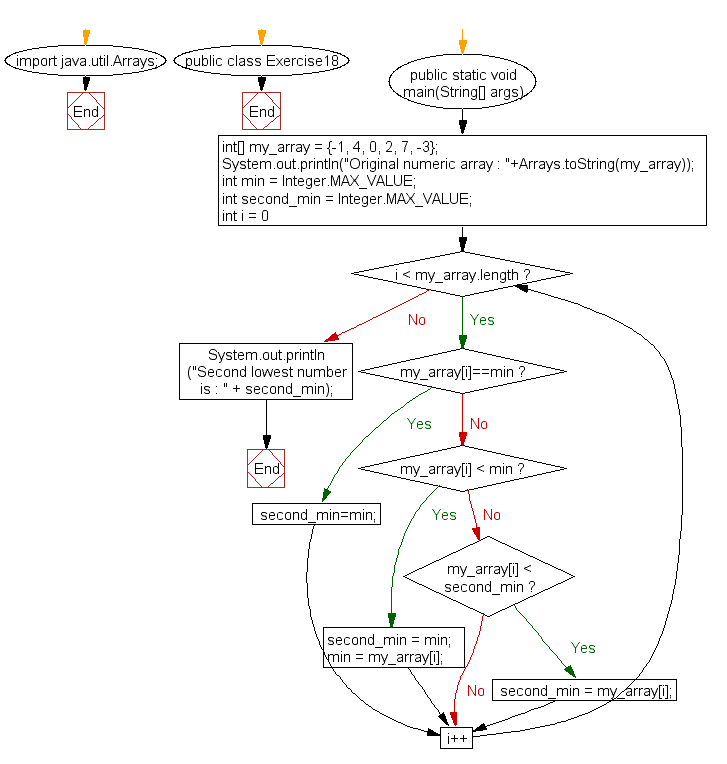﻿ Java exercises: Find the second smallest element in an array - w3resource# Java Array Exercises: Find the second smallest element in an array

## Java Array: Exercise-18 with Solution

Write a Java program to find the second smallest element in an array.

Pictorial Presentation:Sample Solution:

Java Code :

``````import java.util.Arrays;
public class Exercise18 {
public static void main(String[] args) {

int[] my_array = {-1, 4, 0, 2, 7, -3};
System.out.println("Original numeric array : "+Arrays.toString(my_array));
int min = Integer.MAX_VALUE;
int second_min = Integer.MAX_VALUE;
for (int i = 0; i < my_array.length; i++) {
if(my_array[i]==min){
second_min=min;
} else if (my_array[i] < min) {
second_min = min;
min = my_array[i];
} else if (my_array[i] < second_min) {
second_min = my_array[i];
}

}
System.out.println("Second lowest number is : " + second_min);
}
}
```
```

Sample Output:

```Original numeric array : [-1, 4, 0, 2, 7, -3]
Second lowest number is : -1
```

Flowchart:Visualize Java code execution (Python Tutor):

Java Code Editor:

Improve this sample solution and post your code through Disqus

What is the difficulty level of this exercise?

Test your Programming skills with w3resource's quiz.

﻿

## Java: Tips of the Day

countOccurrences

Counts the occurrences of a value in an array.

Use Arrays.stream().filter().count() to count total number of values that equals the specified value.

```public static long countOccurrences(int[] numbers, int value) {
return Arrays.stream(numbers)
.filter(number -> number == value)
.count();
}
```

Ref: https://bit.ly/3kCAgLb